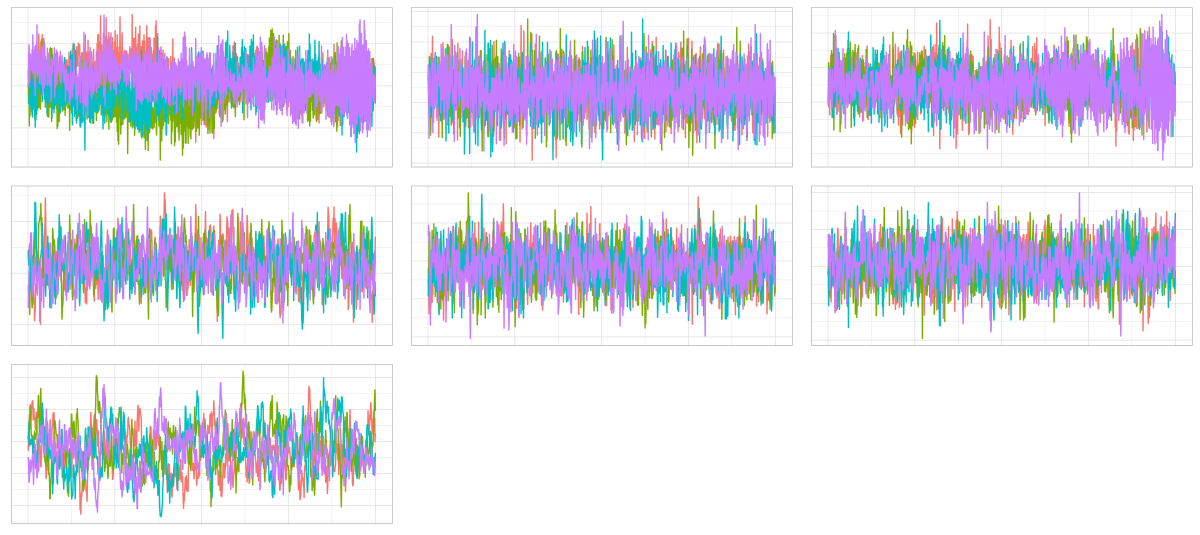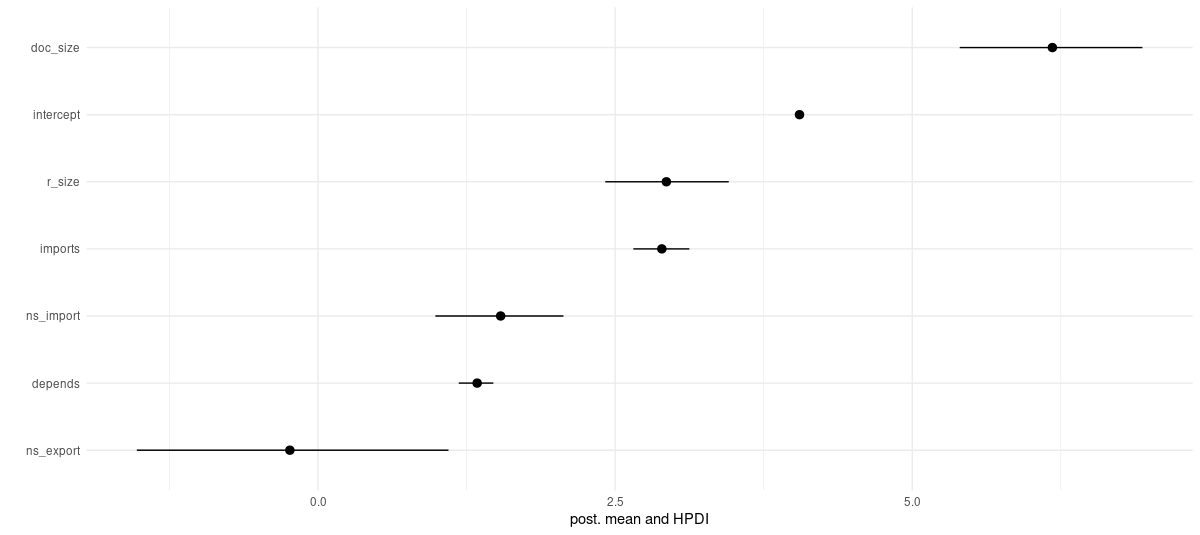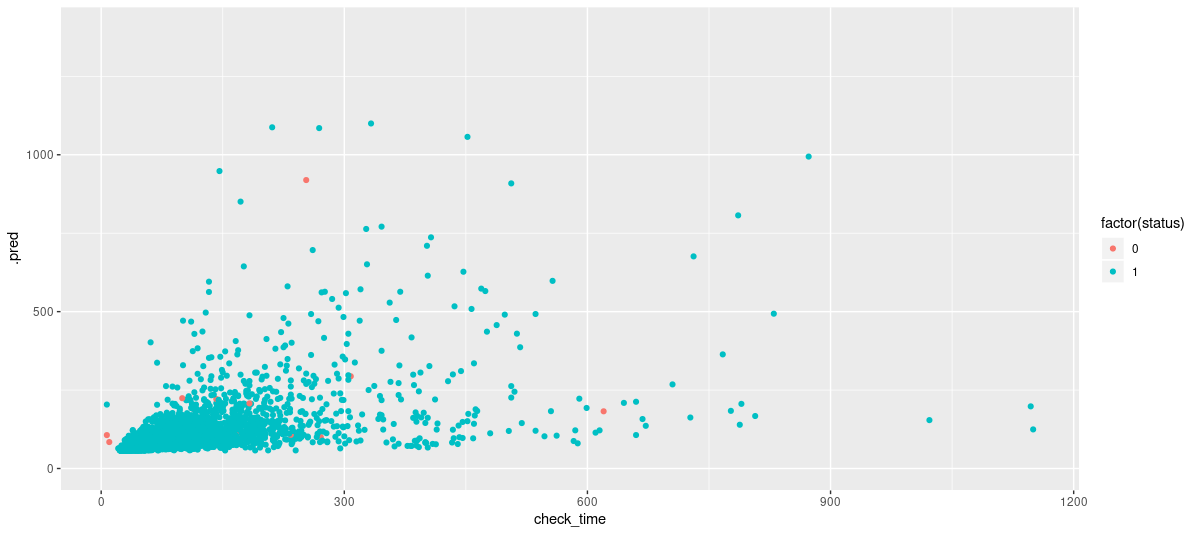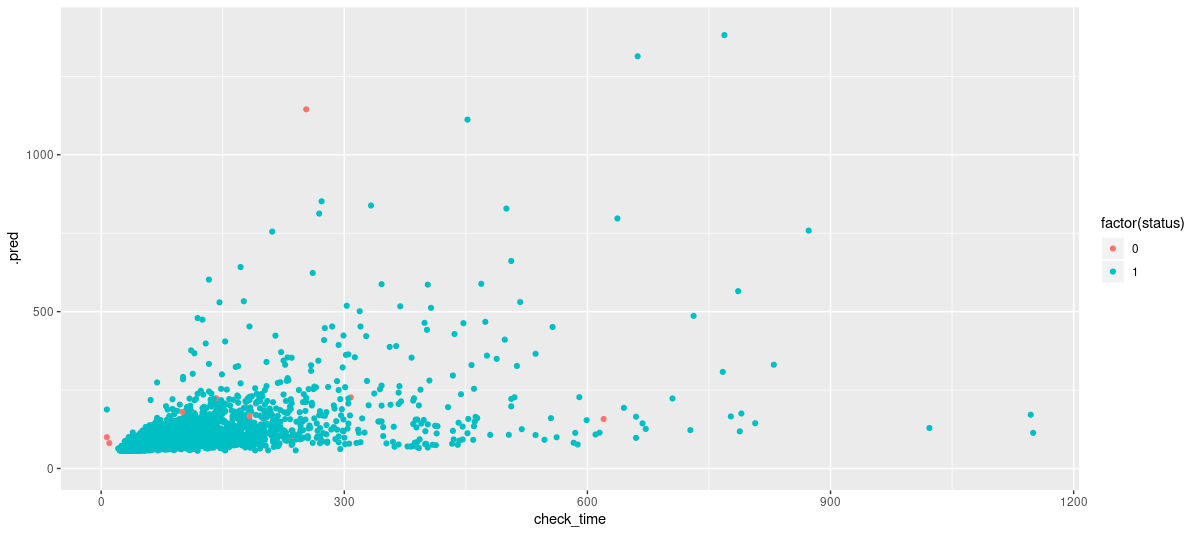# Modeling censored data with tfprobability

In this post we use tfprobability, the R interface to TensorFlow Probability, to model censored data. Again, the exposition is inspired by the treatment of this topic in Richard McElreath’s Statistical Rethinking. Instead of cute cats though, we model immaterial entities from the cold world of technology: This post explores durations of CRAN package checks, a dataset that comes with Max Kuhn’s parsnip.

Sigrid Keydana (RStudio)https://www.rstudio.com/
07-31-2019

Nothing’s ever perfect, and data isn’t either. One type of “imperfection” is missing data, where some features are unobserved for some subjects. (A topic for another post.) Another is censored data, where an event whose characteristics we want to measure does not occur in the observation interval. The example in Richard McElreath’s Statistical Rethinking is time to adoption of cats in an animal shelter. If we fix an interval and observe wait times for those cats that actually did get adopted, our estimate will end up too optimistic: We don’t take into account those cats who weren’t adopted during this interval and thus, would have contributed wait times of length longer than the complete interval.

In this post, we use a slightly less emotional example which nonetheless may be of interest, especially to R package developers: time to completion of `R CMD check`, collected from CRAN and provided by the `parsnip` package as `check_times`. Here, the censored portion are those checks that errored out for whatever reason, i.e., for which the check did not complete.

Why do we care about the censored portion? In the cat adoption scenario, this is pretty obvious: We want to be able to get a realistic estimate for any unknown cat, not just those cats that will turn out to be “lucky”. How about `check_times`? Well, if your submission is one of those that errored out, you still care about how long you wait, so even though their percentage is low (< 1%) we don’t want to simply exclude them. Also, there is the possibility that the failing ones would have taken longer, had they run to completion, due to some intrinsic difference between both groups. Conversely, if failures were random, the longer-running checks would have a greater chance to get hit by an error. So here too, exluding the censored data may result in bias.

How can we model durations for that censored portion, where the “true duration” is unknown? Taking one step back, how can we model durations in general? Making as few assumptions as possible, the maximum entropy distribution for displacements (in space or time) is the exponential. Thus, for the checks that actually did complete, durations are assumed to be exponentially distributed.

For the others, all we know is that in a virtual world where the check completed, it would take at least as long as the given duration. This quantity can be modeled by the exponential complementary cumulative distribution function (CCDF). Why? A cumulative distribution function (CDF) indicates the probability that a value lower or equal to some reference point was reached; e.g., “the probability of durations <= 255 is 0.9”. Its complement, 1 - CDF, then gives the probability that a value will exceed than that reference point.

Let’s see this in action.

## The data

The following code works with the current stable releases of TensorFlow and TensorFlow Probability, which are 1.14 and 0.7, respectively. If you don’t have `tfprobability` installed, get it from Github:

``remotes::install_github("rstudio/tfprobability")``

These are the libraries we need. As of TensorFlow 1.14, we call `tf\$compat\$v2\$enable_v2_behavior()` to run with eager execution.

``````library(tensorflow)
library(tfprobability)
library(parsnip)
library(tidyverse)
library(zeallot)
library(gridExtra)
library(HDInterval)
library(tidymodels)
library(survival)

tf\$compat\$v2\$enable_v2_behavior()``````

Besides the check durations we want to model, `check_times` reports various features of the package in question, such as number of imported packages, number of dependencies, size of code and documentation files, etc. The `status` variable indicates whether the check completed or errored out.

``````df <- check_times %>% select(-package)
glimpse(df)``````
``````Observations: 13,626
Variables: 24
\$ authors        <int> 1, 1, 1, 1, 5, 3, 2, 1, 4, 6, 1, 2, 1, 1, 1, 1, 1, 1, 1, 1,…
\$ imports        <dbl> 0, 6, 0, 0, 3, 1, 0, 4, 0, 7, 0, 0, 0, 0, 3, 2, 14, 2, 2, 0…
\$ suggests       <dbl> 2, 4, 0, 0, 2, 0, 2, 2, 0, 0, 2, 8, 0, 0, 2, 0, 1, 3, 0, 0,…
\$ depends        <dbl> 3, 1, 6, 1, 1, 1, 5, 0, 1, 1, 6, 5, 0, 0, 0, 1, 1, 5, 0, 2,…
\$ Roxygen        <dbl> 0, 1, 0, 0, 1, 0, 0, 1, 0, 0, 1, 0, 1, 0, 1, 0, 1, 1, 1, 0,…
\$ gh             <dbl> 0, 1, 0, 0, 0, 0, 0, 1, 0, 0, 1, 0, 0, 0, 1, 0, 1, 0, 0, 0,…
\$ rforge         <dbl> 0, 0, 0, 0, 0, 0, 0, 0, 0, 0, 0, 0, 0, 0, 0, 0, 0, 0, 0, 0,…
\$ descr          <int> 217, 313, 269, 63, 223, 1031, 135, 344, 204, 335, 104, 163,…
\$ r_count        <int> 2, 20, 8, 0, 10, 10, 16, 3, 6, 14, 16, 4, 1, 1, 11, 5, 7, 1…
\$ r_size         <dbl> 0.029053, 0.046336, 0.078374, 0.000000, 0.019080, 0.032607,…
\$ ns_import      <dbl> 3, 15, 6, 0, 4, 5, 0, 4, 2, 10, 5, 6, 1, 0, 2, 2, 1, 11, 0,…
\$ ns_export      <dbl> 0, 19, 0, 0, 10, 0, 0, 2, 0, 9, 3, 4, 0, 1, 10, 0, 16, 0, 2…
\$ s3_methods     <dbl> 3, 0, 11, 0, 0, 0, 0, 2, 0, 23, 0, 0, 2, 5, 0, 4, 0, 0, 0, …
\$ s4_methods     <dbl> 0, 0, 0, 0, 0, 0, 0, 0, 0, 0, 0, 0, 0, 0, 0, 0, 0, 0, 0, 0,…
\$ doc_count      <int> 0, 3, 1, 0, 1, 0, 0, 0, 0, 0, 0, 0, 0, 0, 1, 0, 0, 0, 0, 0,…
\$ doc_size       <dbl> 0.000000, 0.019757, 0.038281, 0.000000, 0.007874, 0.000000,…
\$ src_count      <int> 0, 0, 0, 0, 0, 0, 0, 2, 0, 5, 3, 0, 0, 0, 0, 0, 0, 54, 0, 0…
\$ src_size       <dbl> 0.000000, 0.000000, 0.000000, 0.000000, 0.000000, 0.000000,…
\$ data_count     <int> 2, 0, 0, 3, 3, 1, 10, 0, 4, 2, 2, 146, 0, 0, 0, 0, 0, 10, 0…
\$ data_size      <dbl> 0.025292, 0.000000, 0.000000, 4.885864, 4.595504, 0.006500,…
\$ testthat_count <int> 0, 8, 0, 0, 0, 0, 0, 1, 0, 0, 0, 0, 0, 0, 0, 0, 3, 3, 0, 0,…
\$ testthat_size  <dbl> 0.000000, 0.002496, 0.000000, 0.000000, 0.000000, 0.000000,…
\$ check_time     <dbl> 49, 101, 292, 21, 103, 46, 78, 91, 47, 196, 200, 169, 45, 2…
\$ status         <dbl> 1, 1, 1, 1, 1, 1, 1, 1, 1, 1, 1, 1, 1, 1, 1, 1, 1, 1, 1, 1,…``````

Of these 13,626 observations, just 103 are censored:

``table(df\$status)``
``````0     1
103 13523 ``````

For better readability, we’ll work with a subset of the columns. We use `surv_reg` to help us find a useful and interesting subset of predictors:

``````survreg_fit <-
surv_reg(dist = "exponential") %>%
set_engine("survreg") %>%
fit(Surv(check_time, status) ~ .,
data = df)
tidy(survreg_fit) ``````
``````# A tibble: 23 x 7
term             estimate std.error statistic  p.value conf.low conf.high
<chr>               <dbl>     <dbl>     <dbl>    <dbl>    <dbl>     <dbl>
1 (Intercept)     3.86      0.0219     176.     0.             NA        NA
2 authors         0.0139    0.00580      2.40   1.65e- 2       NA        NA
3 imports         0.0606    0.00290     20.9    7.49e-97       NA        NA
4 suggests        0.0332    0.00358      9.28   1.73e-20       NA        NA
5 depends         0.118     0.00617     19.1    5.66e-81       NA        NA
6 Roxygen         0.0702    0.0209       3.36   7.87e- 4       NA        NA
7 gh              0.00898   0.0217       0.414  6.79e- 1       NA        NA
8 rforge          0.0232    0.0662       0.351  7.26e- 1       NA        NA
9 descr           0.000138  0.0000337    4.10   4.18e- 5       NA        NA
10 r_count         0.00209   0.000525     3.98   7.03e- 5       NA        NA
11 r_size          0.481     0.0819       5.87   4.28e- 9       NA        NA
12 ns_import       0.00352   0.000896     3.93   8.48e- 5       NA        NA
13 ns_export      -0.00161   0.000308    -5.24   1.57e- 7       NA        NA
14 s3_methods      0.000449  0.000421     1.06   2.87e- 1       NA        NA
15 s4_methods     -0.00154   0.00206     -0.745  4.56e- 1       NA        NA
16 doc_count       0.0739    0.0117       6.33   2.44e-10       NA        NA
17 doc_size        2.86      0.517        5.54   3.08e- 8       NA        NA
18 src_count       0.0122    0.00127      9.58   9.96e-22       NA        NA
19 src_size       -0.0242    0.0181      -1.34   1.82e- 1       NA        NA
20 data_count      0.0000415 0.000980     0.0423 9.66e- 1       NA        NA
21 data_size       0.0217    0.0135       1.61   1.08e- 1       NA        NA
22 testthat_count -0.000128  0.00127     -0.101  9.20e- 1       NA        NA
23 testthat_size   0.0108    0.0139       0.774  4.39e- 1       NA        NA
``````

It seems that if we choose `imports`, `depends`, `r_size`, `doc_size`, `ns_import` and `ns_export` we end up with a mix of (comparatively) powerful predictors from different semantic spaces and of different scales.

Before pruning the dataframe, we save away the target variable. In our model and training setup, it is convenient to have censored and uncensored data stored separately, so here we create two target matrices instead of one:

``````# check times for failed checks
# _c stands for censored
check_time_c <- df %>%
filter(status == 0) %>%
select(check_time) %>%
as.matrix()

# check times for successful checks
check_time_nc <- df %>%
filter(status == 1) %>%
select(check_time) %>%
as.matrix()``````

Now we can zoom in on the variables of interest, setting up one dataframe for the censored data and one for the uncensored data each. All predictors are normalized to avoid overflow during sampling. 1 We add a column of `1`s for use as an intercept.

``````df <- df %>% select(status,
depends,
imports,
doc_size,
r_size,
ns_import,
ns_export) %>%
mutate_at(.vars = 2:7, .funs = function(x) (x - min(x))/(max(x)-min(x))) %>%
add_column(intercept = rep(1, nrow(df)), .before = 1)

# dataframe of predictors for censored data
df_c <- df %>% filter(status == 0) %>% select(-status)
# dataframe of predictors for non-censored data
df_nc <- df %>% filter(status == 1) %>% select(-status)``````

That’s it for preparations. But of course we’re curious. Do check times look different? Do predictors – the ones we chose – look different?

Comparing a few meaningful percentiles for both classes, we see that durations for uncompleted checks are higher than those for completed checks throughout, apart from the 100% percentile. It’s not surprising that given the enormous difference in sample size, maximum duration is higher for completed checks. Otherwise though, doesn’t it look like the errored-out package checks “were going to take longer”?

percentiles: check time 10% 30% 50% 70% 90% 100%
completed 36 54 79 115 211 1343
not completed 42 71 97 143 293 696

How about the predictors? We don’t see any differences for `depends`, the number of package dependencies (apart from, again, the higher maximum reached for packages whose check completed): 2

percentiles: depends 10% 30% 50% 70% 90% 100%
completed 0 1 1 2 4 12
not completed 0 1 1 2 4 7

But for all others, we see the same pattern as reported above for `check_time`. Number of packages imported is higher for censored data at all percentiles besides the maximum:

percentiles: imports 10% 30% 50% 70% 90% 100%
completed 0 0 2 4 9 43
not completed 0 1 5 8 12 22

Same for `ns_export`, the estimated number of exported functions or methods:

percentiles: ns_export 10% 30% 50% 70% 90% 100%
completed 0 1 2 8 26 2547
not completed 0 1 5 13 34 336

As well as for `ns_import`, the estimated number of imported functions or methods:

percentiles: ns_import 10% 30% 50% 70% 90% 100%
completed 0 1 3 6 19 312
not completed 0 2 5 11 23 297

Same pattern for `r_size`, the size on disk of files in the `R` directory:

percentiles: r_size 10% 30% 50% 70% 90% 100%
completed 0.005 0.015 0.031 0.063 0.176 3.746
not completed 0.008 0.019 0.041 0.097 0.217 2.148

And finally, we see it for `doc_size` too, where `doc_size` is the size of `.Rmd` and `.Rnw` files:

percentiles: doc_size 10% 30% 50% 70% 90% 100%
completed 0.000 0.000 0.000 0.000 0.023 0.988
not completed 0.000 0.000 0.000 0.011 0.042 0.114

Given our task at hand – model check durations taking into account uncensored as well as censored data – we won’t dwell on differences between both groups any longer; nonetheless we thought it interesting to relate these numbers.

So now, back to work. We need to create a model.

## The model

As explained in the introduction, for completed checks duration is modeled using an exponential PDF. This is as straightforward as adding tfd_exponential() to the model function, tfd_joint_distribution_sequential(). 3For the censored portion, we need the exponential CCDF. This one is not, as of today, easily added to the model. What we can do though is calculate its value ourselves and add it to the “main” model likelihood. We’ll see this below when discussing sampling; for now it means the model definition ends up straightforward as it only covers the non-censored data. It is made of just the said exponential PDF and priors for the regression parameters.

As for the latter, we use 0-centered, Gaussian priors for all parameters. Standard deviations of 1 turned out to work well. As the priors are all the same, instead of listing a bunch of `tfd_normal`s, we can create them all at once as

``tfd_sample_distribution(tfd_normal(0, 1), sample_shape = 7)``

Mean check time is modeled as an affine combination of the six predictors and the intercept. Here then is the complete model, instantiated using the uncensored data only:

``````model <- function(data) {
tfd_joint_distribution_sequential(
list(
tfd_sample_distribution(tfd_normal(0, 1), sample_shape = 7),
function(betas)
tfd_independent(
tfd_exponential(
rate = 1 / tf\$math\$exp(tf\$transpose(
tf\$matmul(tf\$cast(data, betas\$dtype), tf\$transpose(betas))))),
reinterpreted_batch_ndims = 1)))
}

m <- model(df_nc %>% as.matrix())``````

Always, we test if samples from that model have the expected shapes:

``````samples <- m %>% tfd_sample(2)
samples``````
``````[]
tf.Tensor(
[[ 1.4184642   0.17583323 -0.06547955 -0.2512014   0.1862184  -1.2662812
1.0231884 ]
[-0.52142304 -1.0036682   2.2664437   1.29737     1.1123234   0.3810004
0.1663677 ]], shape=(2, 7), dtype=float32)

[]
tf.Tensor(
[[4.4954767  7.865639   1.8388556  ... 7.914391   2.8485563  3.859719  ]
[1.549662   0.77833986 0.10015647 ... 0.40323067 3.42171    0.69368565]], shape=(2, 13523), dtype=float32)``````

This looks fine: We have a list of length two, one element for each distribution in the model. For both tensors, dimension 1 reflects the batch size (which we arbitrarily set to 2 in this test), while dimension 2 is 7 for the number of normal priors and 13523 for the number of durations predicted.

How likely are these samples?

``m %>% tfd_log_prob(samples)``
``tf.Tensor([-32464.521   -7693.4023], shape=(2,), dtype=float32)``

Here too, the shape is correct, and the values look reasonable.

The next thing to do is define the target we want to optimize.

## Optimization target

Abstractly, the thing to maximize is the log probility of the data – that is, the measured durations – under the model. Now here the data comes in two parts, and the target does as well. First, we have the non-censored data, for which

``m %>% tfd_log_prob(list(betas, tf\$cast(target_nc, betas\$dtype)))``

will calculate the log probability. Second, to obtain log probability for the censored data we write a custom function that calculates the log of the exponential CCDF:

``````get_exponential_lccdf <- function(betas, data, target) {
e <-  tfd_independent(tfd_exponential(rate = 1 / tf\$math\$exp(tf\$transpose(tf\$matmul(
tf\$cast(data, betas\$dtype), tf\$transpose(betas)
)))),
reinterpreted_batch_ndims = 1)
cum_prob <- e %>% tfd_cdf(tf\$cast(target, betas\$dtype))
tf\$math\$log(1 - cum_prob)
}``````

Both parts are combined in a little wrapper function that allows us to compare training including and excluding the censored data. We won’t do that in this post, but you might be interested to do it with your own data, especially if the ratio of censored and uncensored parts is a little less imbalanced.

``````get_log_prob <-
function(target_nc,
censored_data = NULL,
target_c = NULL) {
log_prob <- function(betas) {
log_prob <-
m %>% tfd_log_prob(list(betas, tf\$cast(target_nc, betas\$dtype)))
potential <-
if (!is.null(censored_data) && !is.null(target_c))
get_exponential_lccdf(betas, censored_data, target_c)
else
0
log_prob + potential
}
log_prob
}

log_prob <-
get_log_prob(
check_time_nc %>% tf\$transpose(),
df_c %>% as.matrix(),
check_time_c %>% tf\$transpose()
)``````

## Sampling

With model and target defined, we’re ready to do sampling.

``````n_chains <- 4
n_burnin <- 1000
n_steps <- 1000

# keep track of some diagnostic output, acceptance and step size
trace_fn <- function(state, pkr) {
list(
pkr\$inner_results\$is_accepted,
pkr\$inner_results\$accepted_results\$step_size
)
}

# get shape of initial values
# to start sampling without producing NaNs, we will feed the algorithm
# tf\$zeros_like(initial_betas)
initial_betas <- (m %>% tfd_sample(n_chains))[]``````

For the number of leapfrog steps and the step size, experimentation showed that a combination of 64 / 0.1 yielded reasonable results:

``````hmc <- mcmc_hamiltonian_monte_carlo(
target_log_prob_fn = log_prob,
num_leapfrog_steps = 64,
step_size = 0.1
) %>%

run_mcmc <- function(kernel) {
kernel %>% mcmc_sample_chain(
num_results = n_steps,
num_burnin_steps = n_burnin,
current_state = tf\$ones_like(initial_betas),
trace_fn = trace_fn
)
}

# important for performance: run HMC in graph mode
run_mcmc <- tf_function(run_mcmc)

res <- hmc %>% run_mcmc()
samples <- res\$all_states``````

## Results

Before we inspect the chains, here is a quick look at the proportion of accepted steps and the per-parameter mean step size:

``````accepted <- res\$trace[]
as.numeric(accepted) %>% mean()``````
``0.995``
``````step_size <- res\$trace[]
as.numeric(step_size) %>% mean()``````
``0.004953894``

We also store away effective sample sizes and the rhat metrics for later addition to the synopsis.

``````effective_sample_size <- mcmc_effective_sample_size(samples) %>%
as.matrix() %>%
apply(2, mean)
potential_scale_reduction <- mcmc_potential_scale_reduction(samples) %>%
as.numeric()``````

We then convert the `samples` tensor to an R array for use in postprocessing.

``````# 2-item list, where each item has dim (1000, 4)
samples <- as.array(samples) %>% array_branch(margin = 3)``````

How well did the sampling work? The chains mix well, but for some parameters, autocorrelation is still pretty high.

``````prep_tibble <- function(samples) {
as_tibble(samples,
.name_repair = ~ c("chain_1", "chain_2", "chain_3", "chain_4")) %>%
gather(key = "chain", value = "value",-sample)
}

plot_trace <- function(samples) {
prep_tibble(samples) %>%
ggplot(aes(x = sample, y = value, color = chain)) +
geom_line() +
theme_light() +
theme(
legend.position = "none",
axis.title = element_blank(),
axis.text = element_blank(),
axis.ticks = element_blank()
)
}

plot_traces <- function(samples) {
plots <- purrr::map(samples, plot_trace)
do.call(grid.arrange, plots)
}

plot_traces(samples)``````Figure 1: Trace plots for the 7 parameters.

Now for a synopsis of posterior parameter statistics, including the usual per-parameter sampling indicators effective sample size and rhat.

``````all_samples <- map(samples, as.vector)

means <- map_dbl(all_samples, mean)

sds <- map_dbl(all_samples, sd)

hpdis <- map(all_samples, ~ hdi(.x) %>% t() %>% as_tibble())

summary <- tibble(
mean = means,
sd = sds,
hpdi = hpdis
) %>% unnest() %>%
add_column(param = colnames(df_c), .after = FALSE) %>%
n_effective = effective_sample_size,
rhat = potential_scale_reduction
)

summary``````
``````# A tibble: 7 x 7
param       mean     sd  lower upper n_effective  rhat
<chr>      <dbl>  <dbl>  <dbl> <dbl>       <dbl> <dbl>
1 intercept  4.05  0.0158  4.02   4.08       508.   1.17
2 depends    1.34  0.0732  1.18   1.47      1000    1.00
3 imports    2.89  0.121   2.65   3.12      1000    1.00
4 doc_size   6.18  0.394   5.40   6.94       177.   1.01
5 r_size     2.93  0.266   2.42   3.46       289.   1.00
6 ns_import  1.54  0.274   0.987  2.06       387.   1.00
7 ns_export -0.237 0.675  -1.53   1.10        66.8  1.01``````Figure 2: Posterior means and HPDIs.

From the diagnostics and trace plots, the model seems to work reasonably well, but as there is no straightforward error metric involved, it’s hard to know if actual predictions would even land in an appropriate range.

To make sure they do, we inspect predictions from our model as well as from `surv_reg`. This time, we also split the data into training and test sets. Here first are the predictions from `surv_reg`:

``````train_test_split <- initial_split(check_times, strata = "status")
check_time_train <- training(train_test_split)
check_time_test <- testing(train_test_split)

survreg_fit <-
surv_reg(dist = "exponential") %>%
set_engine("survreg") %>%
fit(Surv(check_time, status) ~ depends + imports + doc_size + r_size +
ns_import + ns_export,
data = check_time_train)
survreg_fit(sr_fit)``````
``````# A tibble: 7 x 7
term         estimate std.error statistic  p.value conf.low conf.high
<chr>           <dbl>     <dbl>     <dbl>    <dbl>    <dbl>     <dbl>
1 (Intercept)  4.05      0.0174     234.    0.             NA        NA
2 depends      0.108     0.00701     15.4   3.40e-53       NA        NA
3 imports      0.0660    0.00327     20.2   1.09e-90       NA        NA
4 doc_size     7.76      0.543       14.3   2.24e-46       NA        NA
5 r_size       0.812     0.0889       9.13  6.94e-20       NA        NA
6 ns_import    0.00501   0.00103      4.85  1.22e- 6       NA        NA
7 ns_export   -0.000212  0.000375    -0.566 5.71e- 1       NA        NA``````
``````survreg_pred <-
predict(survreg_fit, check_time_test) %>%
bind_cols(check_time_test %>% select(check_time, status))

ggplot(survreg_pred, aes(x = check_time, y = .pred, color = factor(status))) +
geom_point() +
coord_cartesian(ylim = c(0, 1400))``````Figure 3: Test set predictions from surv_reg. One outlier (of value 160421) is excluded via coord_cartesian() to avoid distorting the plot.

For the MCMC model, we re-train on just the training set and obtain the parameter summary. The code is analogous to the above and not shown here.

We can now predict on the test set, for simplicity just using the posterior means:

``````df <- check_time_test %>% select(
depends,
imports,
doc_size,
r_size,
ns_import,
ns_export) %>%
add_column(intercept = rep(1, nrow(check_time_test)), .before = 1)

mcmc_pred <- df %>% as.matrix() %*% summary\$mean %>% exp() %>% as.numeric()
mcmc_pred <- check_time_test %>% select(check_time, status) %>%

ggplot(mcmc_pred, aes(x = check_time, y = .pred, color = factor(status))) +
geom_point() +
coord_cartesian(ylim = c(0, 1400)) ``````Figure 4: Test set predictions from the mcmc model. No outliers, just using same scale as above for comparison.

This looks good!

## Wrapup

We’ve shown how to model censored data – or rather, a frequent subtype thereof involving durations – using `tfprobability`. The `check_times` data from `parsnip` were a fun choice, but this modeling technique may be even more useful when censoring is more substantial. Hopefully his post has provided some guidance on how to handle censored data in your own work. Thanks for reading!

1. By itself, the predictors being on different scales isn’t a problem. Here we need to normalize due to the log link used in the model above.↩︎

2. Here and in the following tables, we report the unnormalized, original values as contained in `check_times`.↩︎

3. For a first introduction to MCMC sampling with `tfprobability`, see Tadpoles on TensorFlow: Hierarchical partial pooling with tfprobability↩︎

### Reuse

Text and figures are licensed under Creative Commons Attribution CC BY 4.0. The figures that have been reused from other sources don't fall under this license and can be recognized by a note in their caption: "Figure from ...".

### Citation

`Keydana (2019, July 31). Posit AI Blog: Modeling censored data with tfprobability. Retrieved from https://blogs.rstudio.com/tensorflow/posts/2019-07-31-censored-data/`
```@misc{keydana2019tfpcensored,